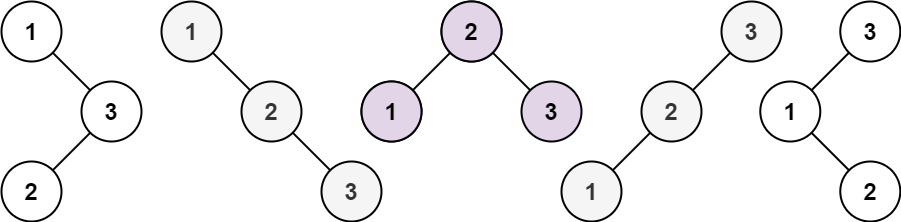## 96. Unique Binary Search Trees

Given an integer `n`, return the number of structurally unique BST's (binary search trees) which has exactly `n` nodes of unique values from `1` to `n`.

Example 1:```Input: n = 3
Output: 5
```

Example 2:

```Input: n = 1
Output: 1
```

Constraints:

• `1 <= n <= 19`

## Rust Solution

``````struct Solution;

impl Solution {
fn num_trees(n: i32) -> i32 {
let n = n as usize;
let mut dp: Vec<i32> = vec![0; n + 1];
dp = 1;
dp = 1;
for i in 2..=n {
for j in 0..i {
dp[i] += dp[j] * dp[i - 1 - j];
}
}
dp[n]
}
}

#[test]
fn test() {
let n = 3;
let res = 5;
assert_eq!(Solution::num_trees(n), res);
}
``````

Having problems with this solution? Click here to submit an issue on github.